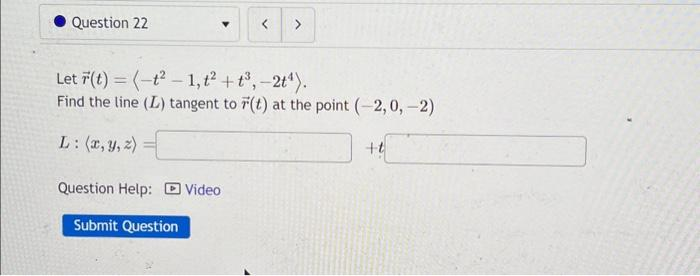Home / Expert Answers / Calculus / question-22-gt-let-r-t-t2-1-t-t-2t-find-the-line-l-tangent-to-r-t-at-the-poin-pa191

# (Solved): Question 22 > Let r(t) = (-t2-1, t + t, -2t). Find the line (L) tangent to r(t) at the poin ...

Question 22 > Let r(t) = (-t2-1, t² + t³, -2t¹). Find the line (L) tangent to r(t) at the point (-2, 0, -2) L: (x, y, z) Question Help: Video Submit Question +t
HELP!Let . Find the line tangent to at the point

We have an Answer from Expert